# Calculation bimetallic actuator

How much does a bimetal actuator bends at a certain temperature and how is this calculated?

### Sketch of a deflected bimetallic actuator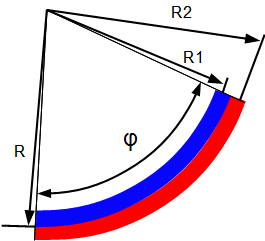A bimetallic element is composed of two materials which are joined together and expand differently with changes in temperature. If the material 2 (shown in red) expands more than when heated material 1 (shown in blue), the material composite curves. The radius of curvature and thus the deflection at the end can be calculated.

Material 1 is blue Material 2 is red. The materials are firmly bonded together.
R: neutral fiber of the bimetallic actuator
R1: neutral fiber of the material 1
R2: neutral fiber of the material 2
A: Coefficient of Thermal Expansion
delta T: temperature difference for hibernation (bimetallic actuator straight)
l0: Length of the bimetallic actuator in the straight state

The length of a metal at a given temperature is: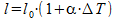Furthermore, the following applies:Change in length of the materials to each other: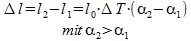Change in length due to the geometry: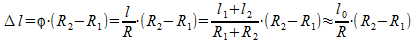Equating the two above formulas gives: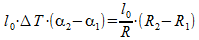After canceling: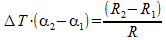Since bimetallic actuators are usually constructed from two materials with the same thickness d remains valid: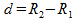it follows: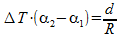Changing according to R.

## The formula for the bimetallic actuator: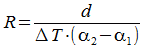Note: This is the minimum bending radius, since the modulus of elasticity of the materials was not taken into account. In reality, the radius of which is slightly larger.

## Example iron-nickel bimetallic actuator: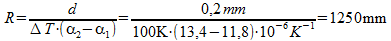Talk to us